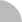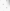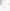##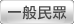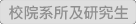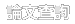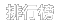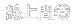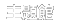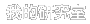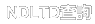(3.238.99.243) 您好！臺灣時間：2021/05/15 18:29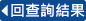### 詳目顯示:::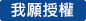: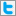Twitter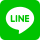•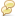被引用:0
•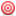點閱:125
•評分: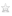•下載:0
•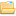書目收藏:0
 令α是界在0和1之間的無理數，且其連分數表示法為[0;a1+1,a2,a3,…]，其中an ≧1 (n≧1)。定義q-1=1, q0=1, qn=anqn-1+qn-2 (n≧1)。對於每一個大於等於-1的整數k，我們考慮雙邊無窮特徵字字尾H的k階分解，形式如下：H=ukuk+1uk+2…，其中因子ui的長度為qi (i≧k)。我們證明在這樣的分解中，不是所有ui皆為奇異字，就是存在非負整數q使得H的前q個因子為奇異字，其餘的皆為α-字。更進一步地，這些α-字的標籤可被一非負整數的F-表現法所唯一決定，此非負整數與H在雙邊無窮特徵字上的開始位置有關。接著，我們定義由一對種子字(u,v)所生成的k階Markov字型(k≧1)，形式如下：Mk(u,v)=z1z2z3…，其中z1=u, z2=v, zi=(zi-1)ai+k-1-1 zi-2 zi-1 (i≧3)。我們證明每一個雙邊無窮特徵字字尾H皆為Markov字型，且其所包含的種子字皆為鄰邊α-字；我們也找到H的所有身為鄰邊α-字的種子字。另一方面，我們證明每一個鄰邊α-字所生成的Markov字型皆為雙邊無窮特徵字字尾。最後，我們研究鄰邊α-字所形成的集合V。我們分別以循環移位、字典順序、標籤描述V中的元素。對於每一個α-字w，我們找到所有α-字v使得(w,v)為一對鄰邊α-字，也找到所有α-字u使得(u,w)為一對鄰邊α-字。另外，我們建造有向圖Gα，此圖的點集為所有α-字所形成的集合，邊集為所有鄰邊α-字所形成的集合；我們以此圖作為例證，說明鄰邊α-字的相關結果，以及它們所生成的雙邊無窮特徵字字尾。
 Let α be an irrational number between 0 and 1 with continued fraction expansion [0;a1+1,a2,a3,…], where an ≧1 (n≧1). Define a sequence of numbers q-1=1, q0=1, qn=anqn-1+qn-2 (n≧1). For each integer k≧-1, we consider the k-order factorization of each suffix H of a two-way infinite characteristic word ofαof the form: H=ukuk+1uk+2…, where the length of the factor ui is qi (i≧k). We show that in such a factorization, either all ui are singular words, or there exists a nonnegative integer q such that H begins with q singular words, and uk+quk+q+1uk+q+2… areα-words. Moreover, the labels of theseα-words are uniquely determined by the F-representation of a nonnegative integer obtained from the position of H in the two-way infinite characteristic word.Next, define Markov word pattern of order k (k≧-1) generated by a pair of seed words (u,v) as follows: Mk(u,v)=z1z2z3…, where z1=u, z2=v, and zi=(zi-1)ai+k-1-1 zi-2 zi-1 (i≧3). We show that each suffix H of each two-way infinite characteristic word is a Markov word pattern, and the pairs of its seed words obtained are adjacentα-words; we also find all possible pairs of seed words of H which are pairs of adjacent α-words. On the other hand, we show that each Markov word pattern generated by any pair of adjacentα-words is a suffix of a two-way infinite characteristic word.Finally, we study the set V of all pairs of adjacentα-words. We describe the elements of V in terms of cyclic shifts, lexicographic order, and labels. For eachα-word w, we find all possible words u such that (u,w) (resp., (w,u)) are pairs of adjacentα-words. Also, we construct a directed graph Gα consisting of the vertex set of allα-words and the edge set V, and use such a graph to illustrate the results about adjacentα-words, and the suffixes of the two-way infinite characteristic words that they generate.
 Contents摘要 IAbstract II誌謝辭 IIIContents IVList of Figures VIList of Tables VIIList of Symbols VIIIChapter 1 Introduction 1Chapter 2 Preliminaries 42.1 Words 42.2 α-words and singular words 52.3 Two-way infinite characteristic words 9Chapter 3 Factorizations of Suffixes of Two-Way Infinite Characteristic Words 123.1 The F-representation of nonnegative integers 133.2 Factorizations of suffixes ofα-words 163.3 (-1)st-order factorization of suffixes of f and f' 243.4 kth-order factorizations (k≧-1) of suffixes of f and f' 333.5 The case α=[0;1+1,1,1,…] 37Chapter 4 Markov Word Patterns in Two-Way Infinite Characteristic Words 404.1 Markov word patterns in f and f' 404.2 More seed words of suffixes of f and f' 45Chapter 5 Adjacentα-Words 505.1 The V+-set 515.1.1 Cyclic shifts 515.1.2 Lexicographic order 535.1.3 Labels 595.2 The V--set 615.3 Suffixes of f and f' determined by a singleα-words 665.4 The set V 675.5 The directed graph Gα 69References 74Appendices 78A.1 Using kth-order factorizations to prove Theorem 4.1.2 78A.2 Using labels in Zn+1 (resp., Zn-1) to rewrite Theorem 5.1.10 (resp., 5.2.5) 79A.3 Using known V+-sets to prove Theorem 5.2.1 81List of FiguresFigure 2.1 The defining tree Tαof α-words with α=[0; a1+1, a2, a3,…]. 7Figure 5.1 The subgraph of Gαwith the elements of W in cyclic shifts andα=[0;3+1,3,1,2,…]. 70Figure 5.2 The subgraph of Gα with the elements of W in lexicographic order andα=[0;3+1,3,1,2,…]. 71Figure 5.3 The directed graph Gα with the elements of W in cyclic shifts andα=[0;3+1,3,1,2,…]. 72Figure 5.4 The directed graph Gα with the elements of W in lexicographic order andα=[0;3+1,3,1,2,…]. 73List of TablesTable 3.1 I(r) for each r in S1∪S2∪S3∪S4 with α=[0;3+1,2,1,2,4,…]. 14Table 3.2 σ( r) for each r in S1∪S2∪S3∪S4 withα=[0;3+1,2,1,2,4,…]. 21Table 3.3 (-1)st-order factorizations of fm and f’m for some m in [-122,28] withα=[0;2+1,2,3,1,1,1,…], where ai=1 for all i≧4. 31Table 3.4 1st-order factorizations of fm and f’m for some m in [-122,28] withα=[0;2+1,2,3,1,1,1,…], where ai=1 for all i≧4. 36Table 4.1 Seed words (in cyclic shifts) of nth-order MWPs in fm, which are pairs of adjacent α-words, where -1≦n≦3 and -9≦m≦100 with α=[0;2+1,2,4,3,2,…]. 47Table 4.2 Seed words (in cyclic shifts) of nth-order MWPs in f’m, which are pairs of adjacent α-words, where -1≦n≦3 and -9≦m≦100 with α=[0;2+1,2,4,3,2,…]. 48Table 5.1 The elements of V+( Ti(xn)) for n≧1 and 0≦i≦q_n-1. 52Table 5.2 The integers i3,k, j3,k, and d3,k for each k in [0, q3-1] withα=[0;3+1,3,1,2,…]. 69
  J. Berstel, P. Seebold, Morphismes de Sturm, B. Belg. Math. Soc. 1 (1994) 175-189. T.C. Brown, A characterization of the quadratic irrationals, Can. Math. Bull. 34 (1991) 36-41. T.C. Brown, Descriptions of the characteristic sequence of an irrational, Can. Math. Bull. 36 (1993) 15-21. W.-T. Cao, Z.-Y. Wen, Some properties of the factors of Sturmian sequences, Theor. Comput. Sci. 304 (2003) 365-385. W.-F. Chuan, Fibonacci words, Fibonacci Quart. 30.1 (1992) 68-76. W.-F. Chuan, Embedding Fibonacci words into Fibonacci word patterns, in: G.E. Bergum, A.N. Philippou, A.F. Horadam (Eds.), Applications of Fibonacci Numbers Vol. 5 (Kluwer, Dordrecht, 1993) 113-122. W.-F. Chuan, Subwords of the golden sequence and the Fibonacci words, in: G.E. Bergum, A.N. Philippou, A.F. Horadam (Eds.), Applications of Fibonacci Numbers, Vol. 6 (Kluwer, Dordrecht, 1996) 73-84. W.-F. Chuan, α-words and factors of characteristic sequences, Discrete Math. 177 (1997) 33-50. W.-F. Chuan, A representation theorem of the suffixes of characteristic sequences, Discrete Appl. Math. 85 (1998) 47-57. W.-F. Chuan, Unbordered factors of the characteristic sequences of irrational numbers, Theor. Comput. Sci. 205 (1998) 337-344. W.-F. Chuan, Sturmian morphisms and α-words, Theor. Comput. Sci. 225 (1999) 129-148. W.-F. Chuan, Characterizations of α-words, moments, and determinants, Fibonacci Quart. 41.3 (2003) 194-208. W.-F. Chuan, C.-H. Chang, Y.-L. Chang, Suffixes of Fibonacci word patterns, Fibonacci Quart. 38.5 (2000) 432-438. W.-F. Chuan, Factors of characteristic words of irrational numbers, Theor. Comput. Sci. 337 (2005) 169-182. W.-F. Chuan, H.-L. Ho, Factors of characteristic words: location and decompositions, Theor. Comput. Sci. 411 (2010) 2827-2846. W.-F. Chuan, H.-L. Ho, Locating factors of a characteristic word via the Zeckendorf representation of numbers, Theor. Comput. Sci. 440-441 (2012) 39-51. W.-F. Chuan, F.-Y. Liao, The D-representation of nonnegative integers and the Fibonacci factorization of suffixes of infinite Fibonacci words, Discrete Appl. Math., to appear. W.-F. Chuan, F.-Y. Liao, H.-L. Ho, F. Yu, Fibonacci word patterns in two-way infinite Fibonacci words, Theor. Comput. Sci. 437 (2012) 69-81. W.-F. Chuan, F. Yu, Three new extraction formulae, Fibonacci Quart. 45.1 (2007) 76-84. A. de Luca, A division property of the Fibonacci word, Inform. Process. Lett. 54 (1995) 307-312. N.P. Fogg, V. Berthe, S. Ferenczi, C. Mauduit, A. Siegel, Substitutions in Dynamics, Arithmetics and Combinatorics, Lecture Notes in Mathematics, Vol. 1794 (2002), Chapter 6. A.S. Fraenkel, Systems of numeration, Am. Math. Mon. 92 (1985) 105-114. A.S. Fraenkel, I. Borosh, A generalization of Wythoff's game, J. Comb. Theory A 15 (1973) 175-191. A.S. Fraenkel, J. Levitt, M. Shimshoni, Characterization of the set of values f=[nα], n=1, 2,…, Discrete Math. 2 (1972) 335-345. Amy Glen, Occurrences of palindromes in characteristic Sturmian words, Theor. Comput. Sci. 352 (2006) 31-46. V.E. Hoggatt, Jr., Fibonacci and Lucas Numbers, New York, Houghton Mifflin Company, 1969. S. Ito and S. Yasutomi, On continued fractions, substitutions and characteristic sequences [nx+y]-[(n-1)x+y], Jpn. J. Math 16 (1990) 287-306. M. Lothaire, Combinatorics on Words, Cambridge Univ. Press, 2002, Chapter 2. G. Melancon, Viennot factorization of infinite words, Inform. Process. Lett. 60 (1996) 53-57. G. Melancon, Lyndon words and singular factors of Sturmian words, Theor. Comput. Sci. 218 (1999) 41-59. G. Melancon, Lyndon factorization of Sturmian words, Discrete Math. 210 (2000) 137-149. H.-J. Shyr, Free Monoids and Languages, Lecture Notes, Institute of Applied Mathematics, National Chung Hsing University, Taichung, Taiwan, ROC (1991). K. Tognetti, G. Winley, T. van Ravenstein, The Fibonacci Tree, Hofstader and the Golden String, in: G.E. Bergum, A.N. Philippou, A.F. Horadam (Eds.), Applications of Fibonacci numbers, Vol.3 (Kluwer, Dordrecht, 1990) 325-334. J.C. Turner, Fibonacci word patterns and binary sequences, Fibonacci Quart. 26.3 (1988) 233-246. J.C. Turner, The alpha and the omega of the Wythoff pairs, Fibonacci Quart. 27.1 (1989) 76-86. B.A. Venkov, Elementary Number Theory, Wolters-Noorhoff, Groningen, 1970. Z.-X. Wen, Z.-Y. Wen, Some properties of the singular words of the Fibonacci word, Eur. J. Combin. 15 (1994) 587-598.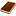國圖紙本論文推文當script無法執行時可按︰推文 網路書籤當script無法執行時可按︰網路書籤 推薦當script無法執行時可按︰推薦 評分當script無法執行時可按︰評分 引用網址當script無法執行時可按︰引用網址 轉寄當script無法執行時可按︰轉寄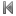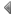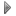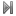top
 無相關論文

 1 王彙中、馬振基，1999，EMI/RFI 遮蔽用導電性高分子複合材料，塑膠資訊，No.33，pp.1~14。 2 王宗雄, 導電高分子聚苯胺之簡介及其應用, 工業材料雜誌, 165, 165-174(2001)

 1 各式弱性微分性質與函數的量度性質 2 以WEIERSTRASS函數座標化表示黎曼最小曲面 3 群組加權之模糊聚類演算法 4 秩序、體嵌入和埃奇勒類數公式 5 三維資料平滑度指標在浮水印的應用 6 影響電腦輔助學習因素之研究 7 質量重分配及其在自旋-1玻色-愛因斯坦凝聚基態上之應用 8 在H空間中極大元與平衡點的存在性定理 9 擬埃爾米特流形上Perelman 的熵公式和海森堡群上的基本定理 10 論多重zeta值的廣義組合等式 11 正特徵值函數體上的逼近指數之研究 12 巴納赫束與測度的擴張 13 南部-泊松M5膜世界體理論及賽伯格威騰映射 14 沿著瑞奇流的κ-noncollapsing估計 15 複單李代數上的有限維對角的自同構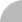簡易查詢 | 進階查詢 | 熱門排行 | 我的研究室